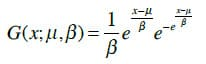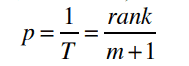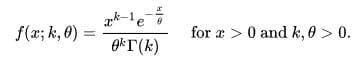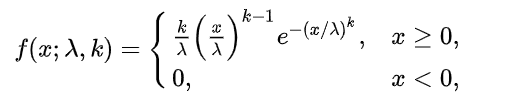## What is rainfall intensity duration frequency (IDF)?

Rainfall Intensity-Duration-Frequency (IDF) is a concept commonly used in hydrology and civil engineering to assess and quantify the rainfall characteristics for a specific location. It helps in understanding the relationship between rainfall intensity, duration, and frequency of occurrence.

Here's what each component means:

• Intensity: Rainfall intensity refers to the rate at which rainwater falls per unit of time. It is typically measured in millimeters per hour (mm/hr) or inches per hour (in/hr). For example, if the intensity is 10 mm/hr, it means that 10 millimeters of rainwater will fall in one hour at that location.
• Duration: Rainfall duration represents the length of time over which the rainfall occurs. It is typically measured in hours. For instance, if the rainfall lasts for 2 hours continuously, the duration is 2 hours.
• Frequency: Rainfall frequency indicates how often a certain intensity and duration of rainfall are expected to occur. It is usually expressed as a return period, often measured in years. For instance, a 50-year rainfall event means the intensity and duration of rainfall are expected to be equaled or exceeded on average once every 50 years.

The IDF relationship is usually derived from historical rainfall data collected over a long period of time for a specific location. Engineers and hydrologists use IDF curves to design stormwater management systems, drainage systems, flood control measures, and other infrastructure to handle rainfall events of different intensities and durations, considering their return periods. These curves are essential for planning and designing resilient and sustainable urban and rural areas.

In hydrology, IDF curves offer a synthetic representation of rainfall events for specific return periods and durations at a given location. These parameters are used to construct synthetic rain graphs and elaborate flood hydrographs, aiding in flood risk assessment and management. Typically, IDF parameters are computed for return periods of 2, 5, 10, 25, 50, and 100 years. This tool aims to calculate IDF curves for the same return periods during past and future periods, providing valuable information for water resources planning and climate change adaptation strategies.

## What is IDF Curve Tool?

The IDF curve tool offers multiple methods for drawing IDF charts and provides two options for inputting data. In the first tab, users can input raw data at different time scales, ranging from 5 minutes to daily observations. The tool then calculates the maximum values in the second tab based on the provided data. Alternatively, users can directly input the maximum values in the second tab, bypassing the need for raw data entry.

In the third tab, users can create IDF graphs for various return periods. A return period represents the average time or estimated average time between rainfall events of a specific intensity. These graphs help in understanding the relationship between rainfall intensity, duration, and frequency.

The tool allows users to save the IDF data in an Excel file, enabling easy access and sharing of results. Additionally, users can draw the IDF graphs directly in Excel for further analysis and visualization.

The IDF curve tool offers users a diverse range of distribution options, Up to now, these distributions are empirical, EV1, Gamma, Weibull, Log-Normal, and Exponential distributions(GEV in the future). Each distribution offers unique modeling approaches to fit the IDF data, enabling users to choose the most appropriate method tailored to their specific requirements and location characteristics. With this flexibility, users can accurately analyze and interpret rainfall intensity, duration, and frequency data, contributing to more effective water resource management and engineering decisions.

### Distributions or Methods

#### Theoretical Extreme Value (EV) distribution

The Theoretical Extreme Value (EV) distribution approach is one of the methods used in calculating Intensity-Duration-Frequency (IDF) curves for rainfall events. It is a statistical approach based on the theory of extreme values, which focuses on the extreme or most significant values in a dataset. This method is particularly useful for modeling rare and extreme rainfall events.where μ is the location parameter and β is the scale parameter.

In the context of IDF curves, the EV distribution approach uses extreme value theory to model the probability distribution of maximum rainfall intensities for different durations and return periods. It assumes that the maximum annual rainfall intensity follows an extreme value distribution.

The key advantage of the EV distribution approach is its ability to provide reliable estimates for extreme rainfall events, even when the available data is limited. It is commonly used in hydrology and water resources engineering for designing structures that can withstand rare and severe rainfall events, such as flood protection systems and stormwater management infrastructure. However, it's worth noting that this approach assumes that extreme events are independent and identically distributed, which may not always hold true in certain cases. Therefore, it's essential to carefully assess the suitability of the EV distribution approach based on the available data and specific application context.

#### Empirical Plotting Position

The Empirical Plotting Position Approach is a statistical method used in hydrology and rainfall analysis to estimate the probability of occurrence of specific rainfall intensities based on observed data. It is commonly used in constructing Intensity-Duration-Frequency (IDF) curves.

The approach involves sorting the rainfall intensity data in ascending order and assigning each observation a plotting position or rank. The plotting position represents the probability of an event exceeding a particular rainfall intensity for a given duration. There are different formulas for calculating plotting positions, and two common methods are the Weibull and Gringorten methods. This tool uses Weibull Empirical Poltting Position:where m is the number of observations, rank is Rank the observations in descending order, p is the exceedance probability and T is the corresponding return period.

#### Gamma Distribution

In the context of Intensity-Duration-Frequency (IDF) calculation, the Gamma distribution is a statistical probability distribution commonly used to model the behavior of rainfall data. It is a continuous probability distribution that has two parameters: shape (k) and scale (θ).Where:

• θ is the scale parameter

• K is the shape parameter

In this tool, we used the Accord libraries to fit the distributions on your data.

#### Weibull Distribution

In the context of Intensity-Duration-Frequency (IDF) calculation, the Weibull distribution is a statistical probability distribution commonly used to model rainfall data. It is a continuous probability distribution that has two parameters: shape (k) and scale (λ).where k > 0 is the shape parameter and λ > 0 is the scale parameter of the distribution.

In this tool, we used the Accord libraries to fit the distributions on your data.

#### Log-Normal and Exponential Distributions

The Log-Normal and Exponential distributions are two commonly used statistical probability distributions in various fields, including hydrology, engineering, finance, and biology. Both distributions have distinct characteristics and applications, making them valuable tools for modeling and analyzing different types of data.

The Log-Normal distribution is a continuous probability distribution characterized by its ability to model positively skewed data. It arises from the logarithm of a random variable following a normal distribution. The Log-Normal distribution is often employed to model variables that are the product of many independent, multiplicative factors. Its applications include modeling extreme events, such as the size of earthquakes, precipitation amounts, and financial returns.

On the other hand, the Exponential distribution is a continuous probability distribution with a simple and straightforward structure. It is commonly used to model time between events in processes that exhibit memoryless behavior, meaning that the probability of an event occurring in a given time interval is constant and does not depend on how much time has elapsed since the last event. This distribution is widely used in reliability analysis, queuing theory, and survival analysis.

In this tool, we used the Accord libraries to fit the distributions on your data.

#### IDF Curve Tool

The license of this tool is applicable for one year of using and you can renew it by pay 20% of the price for the new year.

Subscribe to our Channel In YouTube: